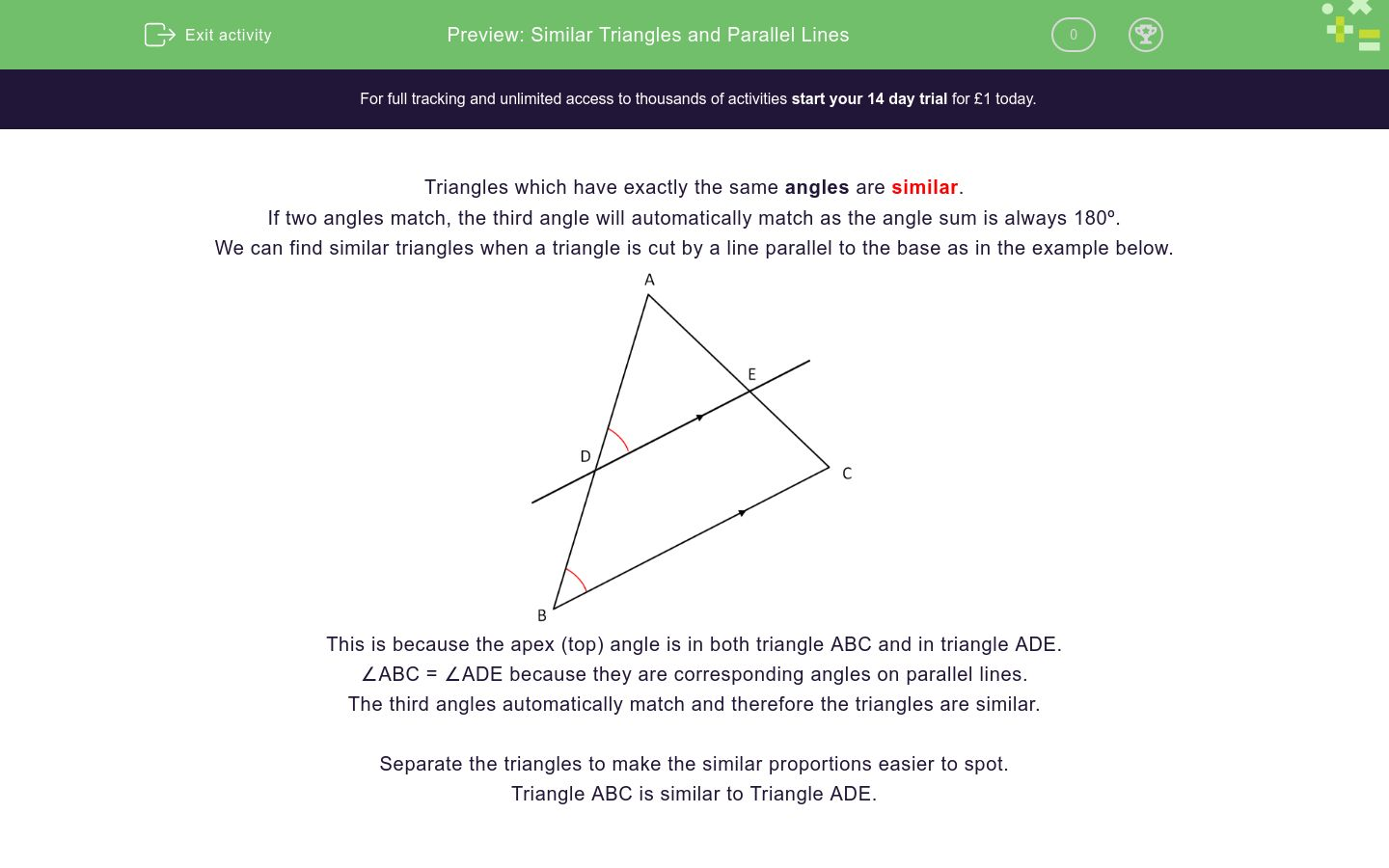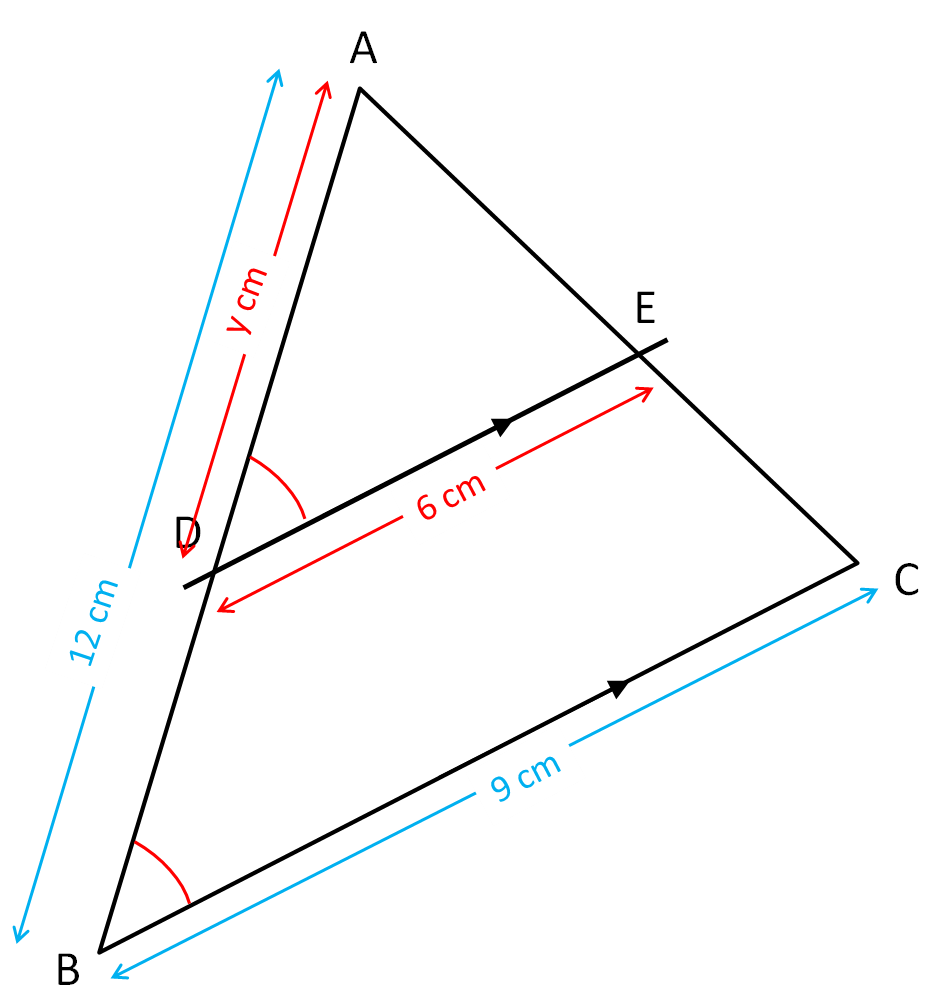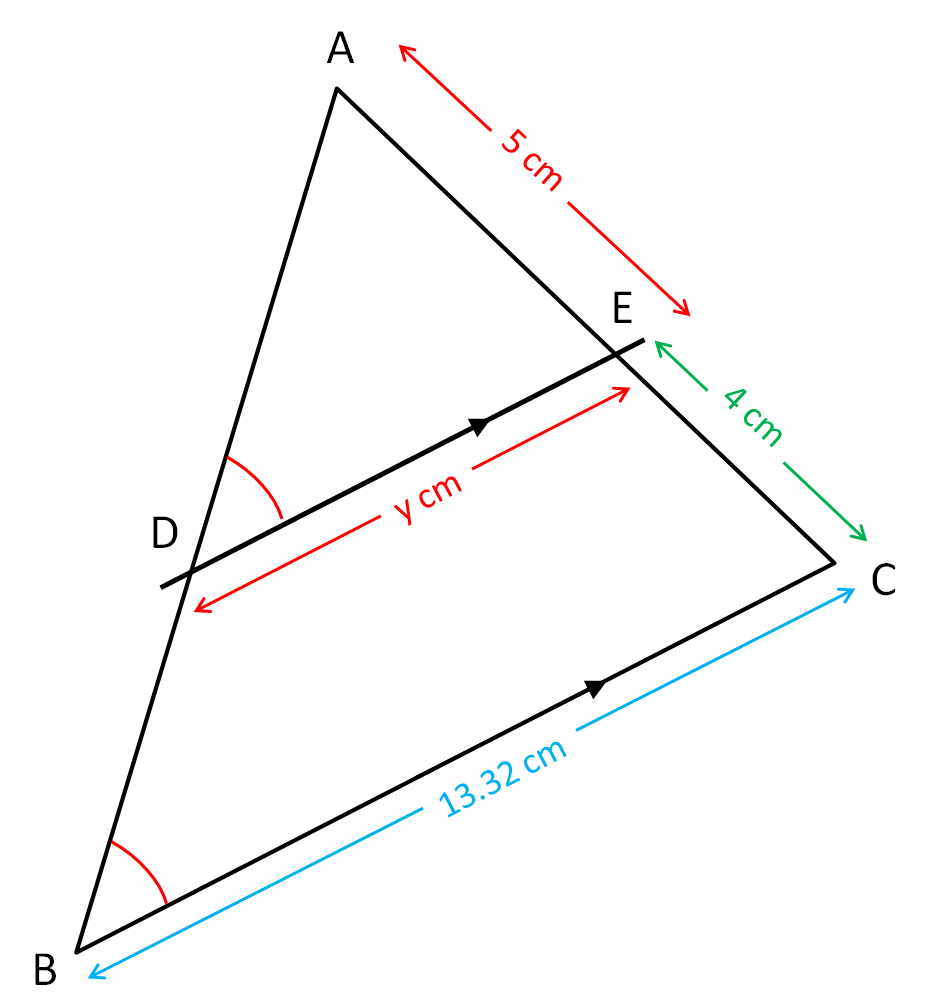# Similar Triangles and Parallel Lines

In this worksheet, students identify similar triangles and use proportions to work out the lengths of sides.Key stage:  KS 4

Curriculum topic:  Geometry and Measures

Difficulty level:### QUESTION 1 of 10

Triangles which have exactly the same angles are similar.

If two angles match, the third angle will automatically match as the angle sum is always 180º.

We can find similar triangles when a triangle is cut by a line parallel to the base as in the example below.This is because the apex (top) angle is in both triangle ABC and in triangle ADE.

∠ABC = ∠ADE because they are corresponding angles on parallel lines.

The third angles automatically match and therefore the triangles are similar.

Separate the triangles to make the similar proportions easier to spot.

Triangle ABC is similar to Triangle ADE.Example

Find the value of y from the information given in this diagram.(not to scale)

The triangles are similar because two angles match, and so will the third too.

Comparing corresponding sides, we get that the scale factor is 5.85 ÷ 4.5 = 1.3

y = 1.3 × 5 = 6.5

Find the value of x from the information given in this diagram.(not drawn to scale)

Find the value of x from the information given in these triangles.(not drawn to scale)

Find the value of y from the information given in this diagram.(not drawn to scale)

Find the value of y from the information given in this diagram.(not drawn to scale)

Find the value of x from the information given in this diagram.(not drawn to scale)

Find the value of y from the information given in this diagram.(not drawn to scale)

Find the value of y from the information given in this diagram.(not drawn to scale)

Find the value of x from the information given in this diagram.(not drawn to scale)

Find the value of x from the information given in this diagram.(not drawn to scale)

Find the value of y from the information given in this diagram.(not drawn to scale)

• Question 1

Find the value of x from the information given in this diagram.(not drawn to scale)

10
EDDIE SAYS
Scale factor = 9 ÷ 4.5 = 2
x = 5 × 2 = 10
• Question 2

Find the value of x from the information given in these triangles.(not drawn to scale)

10.5
EDDIE SAYS
Scale factor = 9 ÷ 6 = 1.5
x = 1.5 × 7 = 10.5
• Question 3

Find the value of y from the information given in this diagram.(not drawn to scale)

8
EDDIE SAYS
Scale factor = 9 ÷ 6 = 1.5
y = 12 ÷ 1.5 = 8
• Question 4

Find the value of y from the information given in this diagram.(not drawn to scale)

9.6
EDDIE SAYS
Scale factor = 7.5 ÷ 6 = 1.25
y = 12 ÷ 1.25 = 9.6
• Question 5

Find the value of x from the information given in this diagram.(not drawn to scale)

9.45
EDDIE SAYS
Scale factor = 8.1 ÷ 6 = 1.35
x = 7 × 1.35 = 9.45
• Question 6

Find the value of y from the information given in this diagram.(not drawn to scale)

8.2
EDDIE SAYS
Scale factor = 8.1 ÷ 6 = 1.35
y = 11.07 ÷ 1.35 = 8.2
• Question 7

Find the value of y from the information given in this diagram.(not drawn to scale)

7.2
EDDIE SAYS
Scale factor = 7.83 ÷ 5.4 = 1.45
y = 10.44 ÷ 1.45 = 7.2
• Question 8

Find the value of x from the information given in this diagram.(not drawn to scale)

11.658
EDDIE SAYS
Scale factor = 7.83 ÷ 5.4 = 1.45
x = 8.04 × 1.45 = 11.658
• Question 9

Find the value of x from the information given in this diagram.(not drawn to scale)

12
EDDIE SAYS
Scale factor = (6 + 3) ÷ 6 = 1.5
x = 8 × 1.5 = 12
• Question 10

Find the value of y from the information given in this diagram.(not drawn to scale)

7.4
EDDIE SAYS
Scale factor = (5 + 4) ÷ 5 = 1.8
y = 13.32 ÷ 1.8 = 7.4
---- OR ----

Sign up for a £1 trial so you can track and measure your child's progress on this activity.

### What is EdPlace?

We're your National Curriculum aligned online education content provider helping each child succeed in English, maths and science from year 1 to GCSE. With an EdPlace account you’ll be able to track and measure progress, helping each child achieve their best. We build confidence and attainment by personalising each child’s learning at a level that suits them.

Start your £1 trial